Next: Spectral capability of the Up: Flux conversions Previous: Count rate predictions for

## Count rate predictions for model atmosphere spectra

The model atmospheres (the atmospheres were computed by M.A.Barstow using computer code originally developed by G.A.Williams, J.Brooker and A.R.King) used here are:

• (i) a pure H-atmosphere with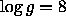;
• (ii) a He-atmosphere with trace H ([He]:[H]=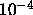) and;

Models (i) and (ii) are representative models for hot DA white dwarfs (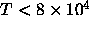K) with different compositions. Care should always be taken when interpreting the results given in Figures 12.6and 12.7since XUV fluxes are extremely sensitive to He abundances which can differ markedly from these models in individual objects.

For each model atmosphere, and for each of the scientific filters as listed in Table 5.3, Figures 12.6and 12.7show the expected WFC count rate as a function of temperature for a set of column densities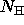varying from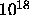tocm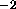. For these model atmospheres the plots are normalized to a V-magnitude, thus the estimated WFC count rate can be determined simply by scaling the conversion factor (CF) to the V-magnitude of the source in question,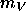, i.e.,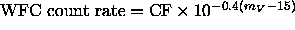.

Michael Arida
Tue Jun 11 16:18:41 EDT 1996